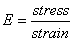# Elastic Modulus

Elastic modulus is sometimes called Young’s modulus after Thomas Young who published the concept back in 1807. An elastic modulus (E) can be determined for any solid material and represents a constant ratio of stress and strain (a stiffness):A material is elastic if it is able to return to its original shape or size immediately after being stretched or squeezed. Almost all materials are elastic to some degree as long as the applied load does not cause it to deform permanently. Thus, the “flexibility” of any object or structure depends on its elastic modulus and geometric shape.

The modulus of elasticity for a material is basically the slope of its stress-strain plot within the elastic range (as shown in Figure 1). Figure 2 shows a stress versus strain curve for steel. The initial straight-line portion of the curve is the elastic range for the steel. If the material is loaded to any value of stress in this part of the curve, it will return to its original shape. Thus, the modulus of elasticity is the slope of this part of the curve and is equal to about 207,000 MPa (30,000,000 psi) for steel. It is important to remember that a measure of a material’s modulus of elasticity is not a measure of strength. Strength is the stress needed to break or rupture a material (as illustrated in Figure 1), whereas elasticity is a measure of how well a material returns to its original shape and size.

### Nomenclature and Symbols

The nomenclature and symbols from the 1993 AASHTO Guide is generally used in referring to pavement moduli. For example:

• EAC = asphalt concrete elastic modulus
• E BS = base course resilient modulus
• ESB = subbase course resilient modulus
• MR (or ESG) = roadbed soil (subgrade) resilient modulus (used interchangeably)

### Stress Sensitivity of Moduli

Changes in stress can have a large impact on resilient modulus. “Typical” relationships are shown in Figures 3 and 4.